#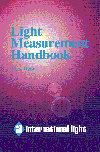Basic Principles

## The Inverse Square Law

The inverse square law defines the relationship between the irradiance from a point source and distance.  It states that the intensity per unit area varies in inverse proportion to the square of the distance.
E = I / d2

In other words, if you measure 16 W/cm2 at 1 meter, you will measure 4 W/cm2 at 2 meters, and can calculate the irradiance at any other distance.  An alternate form is often more convenient:

E1 d12 = E2 d22

Distance is measured to the first luminating surface - the filament of a clear bulb, or the glass envelope of a frosted bulb.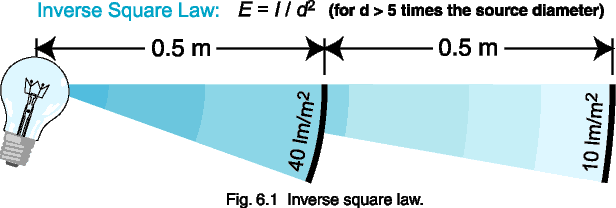### Example:

You measure 10.0 lm/m2 from a light bulb at 1.0 meter.  What will the flux density be at half the distance?

### Solution:

E1 = (d2 / d1)2 * E2
E0.5 m = (1.0 / 0.5)2 * 10.0 = 40 lm/m2

## Point Source Approximation

The inverse square law can only be used in cases where the light source approximates a point source.  A general rule of thumb to use for irradiance measurements is the five times rule:  the distance to a light source should be greater than five times the largest dimension of the source.  For a clear enveloped lamp, this may be the length of the filament.  For a frosted light bulb, the diameter is the largest dimension.  Figure 6.2 below shows the relationship between irradiance and the ratio of distance to source radius.  Note that for a distance 10 times the source radius (5 times the diameter), the error from using the inverse square is exactly 1 %, hence the five times approximation.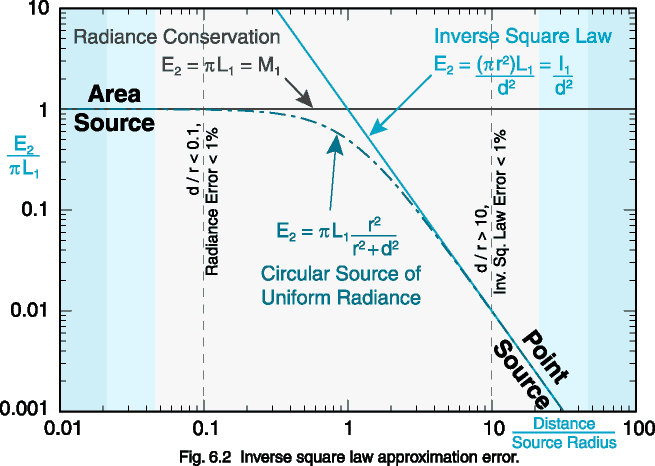Note also, that when the ratio of distance to source radius decreases to below 0.1 (1/20 the diameter of the source), changes in distance hardly affect the irradiance (< 1 % error).  This is due to the fact that as the distance from the source decreases, the detector sees less area, counteracting the inverse square law.  The graph above assumes a cosine response.  Radiance detectors restrict the field of view so that the d/r ratio is always low, providing measurements independent of distance.

## Lamberts Cosine Law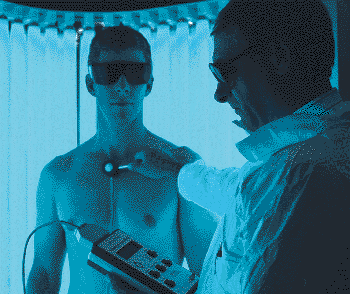The irradiance or illuminance falling on any surface varies as the cosine of the incident angle, q.  The perceived measurement area orthagonal to the incident flux is reduced at oblique angles, causing light to spread out over a wider area than it would if perpendicular to the measurement plane.

To measure the amount of light falling on human skin, you need to mimic the skins cosine response.  Since filter rings restrict off-angle light, a cosine diffuser must be used to correct the spatial responsivity.  In full immersion applications like the phototherapy booth shown above, off angle light is significant, requiring accurate cosine correction optics.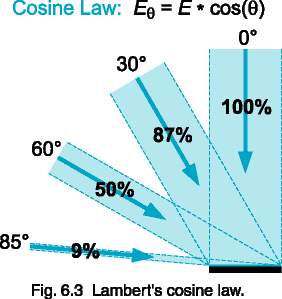## Lambertian Surface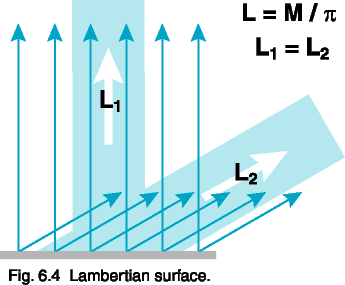A Lambertian surface provides uniform diffusion of the incident radiation such that its radiance or luminance is the same in all directions from which it can be measured.  Many diffuse surfaces are, in fact, Lambertian.  If you view this Light Measurement Handbook from an oblique angle, it should look as bright as it did when held perpendicular to your line of vision.  The human eye, with its restricted solid viewing angle, is an ideal luminance, or brightness, detector.

Figure 6.4 shows a surface radiating equally at 0° and at 60°.  Since, by the cosine law, a radiance detector sees twice as much surface area in the same solid angle for the 60° case, the average incremental reflection must be half the magnitude of the reflection in the 0° case.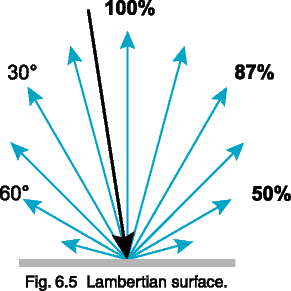Figure 6.5 shows that a reflection from a diffuse Lambertian surface obeys the cosine law by distributing reflected energy in proportion to the cosine of the reflected angle.

A Lambertian surface that has a radiance of 1.0 W/cm2/sr will radiate a total of p*A watts, where A is the area of the surface, into a hemisphere of 2p steradians.  Since the radiant exitance of the surface is equal to the total power divided by the total area, the radiant exitance is p W/cm2.  In other words, if you were to illuminate a surface with an irradiance of 3.1416 W/cm2, then you will measure a radiance on that surface of 1.00 W/cm2/sr (if it is 100% reflective).

The next section goes into converting between measurement geometries in much greater depth.

## Next

### Contents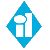HOME